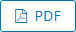вул. Інститутська 11, м. Хмельницький, 29016

МЕТОД КЛАСТЕРИЗАЦІЇ ДАНИХ НА ОСНОВІ АЛГОРИТМУ ОБХОДУ ГРАФА

CLUSTERIZATION METHOD BASED ON BREADTH FIRST SEARCH OR BFS FOR A GRAPH

Сторінки: 8791. Номер: №2, 2022 (307)Автори:
ПЕТРОВ Д. Д.
https://orcid.org/0000-0001-9108-7903
e-mail: dmytro.petrov.knm.2018@lpnu.ua
БОЙЧУК А. Р.
Національний університет “Львівська Політехніка”
https://orcid.org/0000-0002-0563-5748
e-mail: andrii.r.boichuk@lpnu.ua
PETROV DMYTRO D., BOICHUK ANDRYY R.
Lviv Polytechnic National University
DOI: https://www.doi.org/10.31891/2307-5732-2022-307-2-87-91

Анотація мовою оригіналу

В роботі наведено результати досліджень методу кластеризації побудованому на основі обходу графу у ширину та представлено результати роботи на типовому наборі даних для задачі кластеризації, проведено порівняльний аналіз застосування методу.
Ключові слова: кластеризація, граф, обхід графа в ширину.

Розширена анотація англійською  мовою

Clusterization is one of the types of algorithms of unsupervised learning. The idea behind it is that an algorithm learns patterns from untagged data. Such type of algorithm helps to find unseen dependencies in the untagged data itself. This paper presented algorithms based on Breadth-First Search or BFS for a Graph. The method was built based on the basic theory of clusterization. To the theory of clusterization, the calculated distance between the two farthest points in the cluster should be less than the distance between the closest two points from different clusters. By this rule, we defined that two parameters of the method should be the maximum distance between points by which these can be connected and assumed to be in one cluster. The second had to be the maximum distance in the cluster, aka the cluster’s diameter. A cluster’s diameter is the farthest distance between two points within a cluster. With these hyperparameters and the defined distance method, we can assume that every point is a vertex of a graph, two points within the threshold of the distance between pairs of ones are neighbours, and count the connection between counts as an edge of a graph. The group of connected vertexes or a particular vertex is a graph. The diameter hyperparameter ought to keep the data homogeneity in a cluster. We can define every graph as a cluster with defined rules based on previous assumptions. Later in this paper will be visualized the clusterization of three-dimensional data points. We took one of the most popular clusterization dataset – the iris dataset for visualizing purposes. The paper contains several examples of clusterization of the dataset with different hyperparameters. We took KMeans  as an example of the clusterization method. The method based on BFS is a flexible clusterization method that relies on meta-information about distancing between data points.
Keywords: clusterization, BFS, KMeans, unsupervised. .

Література

1. Tan P.-N., Steinbach M., Kumar V. Data mining cluster analysis: basic concepts and algorithms // Introduction to data mining. Pearson Education India, 2013. Vol. 487. P. 533.
2. Kurant M., Markopoulou A., Thiran P. On the bias of BFS (breadth first search) // 2010 22nd International Teletraffic Congress (lTC 22). IEEE, 2010. P. 1–8.
3. Likas A., Vlassis N., Verbeek J.J. The global k-means clustering algorithm // Pattern recognition. Elsevier, 2003. Vol. 36, № 2. P. 451–461.
4. Barlow H.B. Unsupervised learning // Neural computation. MIT Press One Rogers Street, Cambridge, MA 02142-1209, USA journals-info …, 1989. Vol. 1, № 3. P. 295–311.
5. West D.B. Introduction to graph theory. Prentice hall Upper Saddle River, 2001. Vol. 2.
6. Gower J.C. Properties of Euclidean and non-Euclidean distance matrices // Linear algebra and its applications. Elsevier, 1985. Vol. 67. P. 81–97.
7. Hoey P.S. Statistical Analysis of the Iris Flower Dataset // University of Massachusetts. 2004.
8. Pantone color list · toolstud.io [Electronic resource]. URL: https://toolstud.io/color/pantone.php (accessed: 13.04.2022).

References

1. Tan P.-N., Steinbach M., Kumar V. Data mining cluster analysis: basic concepts and algorithms // Introduction to data mining. Pearson Education India, 2013. Vol. 487. P. 533.
2. Kurant M., Markopoulou A., Thiran P. On the bias of BFS (breadth first search) // 2010 22nd International Teletraffic Congress (lTC 22). IEEE, 2010. P. 1–8.
3. Likas A., Vlassis N., Verbeek J.J. The global k-means clustering algorithm // Pattern recognition. Elsevier, 2003. Vol. 36, № 2. P. 451–461.
4. Barlow H.B. Unsupervised learning // Neural computation. MIT Press One Rogers Street, Cambridge, MA 02142-1209, USA journals-info …, 1989. Vol. 1, № 3. P. 295–311.
5. West D.B. Introduction to graph theory. Prentice hall Upper Saddle River, 2001. Vol. 2.
6. Gower J.C. Properties of Euclidean and non-Euclidean distance matrices // Linear algebra and its applications. Elsevier, 1985. Vol. 67. P. 81–97.
7. Hoey P.S. Statistical Analysis of the Iris Flower Dataset // University of Massachusetts. 2004.

Translate